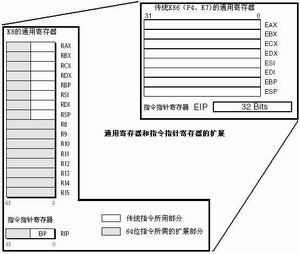# 优化程序性能（读书笔记）

1.gcc -o1 gcc -o2 使程序全面优化

2.如果编译器不能确定两个指针是否指向同一个位置，就必须假设什么情况都可能发生限制了可能的优化策略。

3.对于++ — 函数，内联函数优化函数调用

######4.在for（）中尽量减少函数调用。在里面减少使用strlen（） 这种使用 。尽量在loop前就将这些存放在临时变量里面。

__6.对于=，尽量在for（）之前就存储，在loop之后存储在P里面 。要知道从*p中取值存值耗费指令。__

7.现代处理器中，多级流水线 ICU and EU 分支预测

8.寄存器重命名9.乱序执行

1. R1=M
2. R1=R1+2
3. M=R1
4. R1=M
5. R1=R1+4
6. M=R1 第4、第5、第6条指令在功能上是不依赖于第1、第2、第3条指令的。但是处理器却不能在第3条指令完成前去完成第4条指令（在指令流水线上，不能在第3条指令完成前，就提交第4条指令的结果），因为这可能会导致第3条指令把错误的数据写入内存。

1. R1=M 4. R2=M
2. R1=R1+2 5. R2=R2+4
3. M=R1 6. M=R2 现在，4、5、6号指令可以与1、2、3号指令并行执行，二者没有依赖问题。这使得程序可以用更短时间完成。

10.程序指令在CPU中执行的时候，为了加快执行速度引入流水线技术。流水线上的每一步都执行其中的一条指令。不同类型的数据在进行运算时延迟不同。int类型的加法乘法延迟明显小于单精度float与多精度double的延迟。所以我们在优化一些代码的时候，可以进行分解，使得不同的代码分支并行的运行在多核环境中。

11.CPE叫做每元素的周期数。用来刻画每个元素的周期数而不是每次循环的周期数。看个汇编代码的例子

``````
.L488
mulss (%rax,%rdx,4),%xmm0
compq %rdx,%rbp
jg .L488
``````

``````
int i=0;
for(i=0;i< limit;i+=2)
{
acc0 = acc0 * data[i];
acc1 = acc1 * data[i+1];
}
for(;i< length;i++)
{
acc0 = acc0 *data[i];
}
``````

#####大多数gcc使用 -o1 -o2 只会对整形变量进行优化重新组合，对于浮点型不会做重新组合。 下面考虑这五种形式的loop计算

``````
for(i=0;i< n-2;i+=3)
{
x = a[i];y= a[i+1];z=a[a+2];
r = ((r * x) * y) *z ;  //A1
r = (r * (x * y)) *z ;   //A2
r = r * ((x * y) *z) ;  //A3
r = r * (x * (y * z));  //A4
r = (r * x) * (y *z );  //A5
}
``````

A1每次都要进行乘法，则CPE = 5 * 3/3 = 5 。 A2中r需要两次乘法一次是xy ，另一次是 z ，则CPE = 52 /3 = 3.33 。 A3中r只有在最后一次才会用到，也就是1.67。 A4也是最后一次 ，也是1.67 。 A5需要两次 一次是x 另一次是y*z CPE是3.33 。 可以看出不同的结合顺序，使得CPE值变化较大！